### IMO Shortlist 1974 problem 1

Kvaliteta:
Avg: 0,0
Težina:
Avg: 0,0
Three players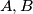$A,B$ and$C$ play a game with three cards and on each of these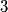$3$ cards it is written a positive integer, all$3$ numbers are different. A game consists of shuffling the cards, giving each player a card and each player is attributed a number of points equal to the number written on the card and then they give the cards back. After a number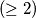$(\geq 2)$ of games we find out that A has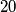$20$ points,$B$ has$10$ points and$C$ has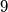$9$ points. We also know that in the last game B had the card with the biggest number. Who had in the first game the card with the second value (this means the middle card concerning its value).
Izvor: Međunarodna matematička olimpijada, shortlist 1974